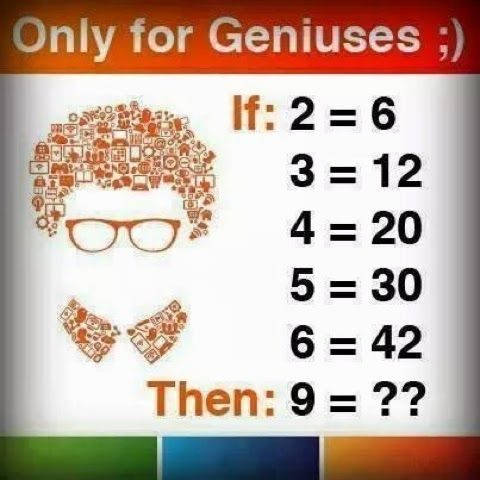This post may contain affiliate links. This means if you click on the link and purchase the item, We will receive an affiliate commission at no extra cost to you. See Our Affiliate Policy for more info.

If 2 = 6 then 9 = ?? – Solve Math Puzzles – Only for Genius Puzzles Images

1
5637

Solve the Series Number Puzzles. Can you solve this Only for Genius Math Puzzles?

Solve this simple math puzzle. All you have to do is just, find the trick and then apply it to the last number i.e 9 =??. Let see if find the answer for this simple logic puzzle. Check puzzle question and image below.

Question: If 2 = 6, 3 = 12, 4 = 20, 5 = 30, 6 = 42, Then 9 = ??Consider, if the number is ‘A’, then Multiply the number A with ‘A+1’  i.e.  A x (A+1) = __

Like given 2=6, it’s mean 2 x (2+1) = 6, or simply 2 x 3 = 6

for next number 3, it is 3 x 4 =12 and so on..

Don’t forget, number 7 and 8

So, overall calculation is shown blow:

2 x 3 =6

3 x 4 =12

4 x 5 = 20

5  x 6 = 30

6 x 7 = 42

7 x 8 = 56 (skipped in image)

8 x 9 = 72 (skipped in image)

9 x 10 = 90

Share if you are right and reply this link to your friends, and find out if they can solve this puzzle or not.

Lets us know your thought or have different method via comments and don’t forget to share!

Search items:

Maths Puzzle Games, Maths Puzzles Images, Puzzles for Genius, Puzzles Games, Maths Problems, Math Puzzles, Only for Genius, Puzzles for Genius, Genius Puzzles Question Image, Logic Puzzles, Math Puzzles Riddles, Difficult Math Puzzles, Only for Genius Puzzles, Math Puzzles with Answer, Logic Puzzles question, Puzzles Question Answer, Puzzles for Facebook, Puzzles for Whatsapp, Puzzles Images for Whatsapp, WhatsApp Puzzles Images, Share Puzzles Images,

1.bookworm1102